Related Articles

# Rational Numbers: Definition, Properties and Examples

Rational Numbers are the most fundamental subset of the collection of all numbers. A rational number represents the fraction of two integers where the denominator can’t be 0. In various applications, throughout the different streams of mathematics rational numbers and their properties are used such as number theory, algebra, calculus, etc. In this article, we will explore rational numbers in depth with various examples, properties, and operations that we can operate on the collection of rational numbers.

## What is a Rational Number?

Rational numbers can be defined as numbers represented in the form of p/q where p and q are both integers and q ≠ 0. Also, any fraction where the denominator is not equal to zero is considered to be a rational number. Thus, rational numbers are a group of numbers that includes fractions, decimals, whole numbers, and natural numbers, also the collection of all rational numbers is denoted by Q.

• All natural numbers, whole numbers, integers, and fractions are rational numbers.
• Every rational number can be represented on a number line.
• 0 is neither a positive nor a negative rational number.

### Rational Number Definiton

A number in the form of p/q where p and q are integers and q ≠ 0 is called a Rational Number. For Example, 2/3, -3/4, 22/7 etc.

### Examples of Rational Numbers

Various types of numbers come under rational numbers. Some examples of rational numbers are as follows:

• 0 (which can be written as 0/1)
• 19  (which can be written as 19/1)
• 2/9
• √(64) which gives 4 or 4/1
• -6/7
• 0.333333 = 1/3
• -0.9 = -9/10

Curious about the nature of π? Read – Is π a rational or irrational number?

### Rational Number Set

In Set Theory, Rational Number is represented as Q. If Q is a set of Rational Numbers then set of Natural Numbers (N), Whole Numbers (W) and Integers (Z) are all subsets of Rational Number Set.

## How to Identify Rational Numbers?

All the rational numbers follow the following rules, thus using the help of these rules we can identify the rational numbers

• Rational numbers are represented in the form of p/q, where q≠0.
• Ratio p/q can be further simplified in simple form or decimal expansion.
• Non-terminating decimals with repeating decimal values are also considered rational numbers as they can be represented in the form of p/q.

Example: Which of the following numbers are rational numbers?

a) -1.75
b) 2/3
c) √5
d) π

Solution:

a) -1.75 is a rational number as it  it has a terminating decimal expansion.

b) 2/3 is also a rational number as it can be expressed in the form of a ratio of two integers.

c) √5 is an irrational number because  it has a decimal expansion with infinitly many digits without any repetation.

d) π is also an irrational number as it has a decimal expansion with infinitly many digits without any repetation.

Thus, only (a) and (b) are the rational numbers out of all the given numbers.

## Types of Rational Numbers

Various types of numbers can be represented as rational numbers some of which are discussed below:

Integers: All the integers, i.e. negative integers and positive integers both come under rational numbers. For example, -1, -11, -4, 6. 8. 10. etc all are rational numbers.

As natural numbers, whole numbers, and others come under rational numbers they are all rational numbers.

Fraction Number: A rational number is a ratio of two integers that can be written in the form of p/q where q is not equal to zero. Hence, any fraction with a non-zero denominator is a rational number.

Example: -2 / 5 is a rational number where -2 is an integer being divided by a non-zero integer 5

Decimal Number: A rational number can be also written in the decimal form if the decimal value is definite or has repeating digits after the decimal point.

Example: 0.3 is a rational number. The value 0.3 can be further expressed in the form of a ratio or fraction as p/q

0.3 = 3/10

Also, 1.333333… can be represented as 4/3 hence, 1.33333… is a rational number.

The image added below shows types of Rational Numbers.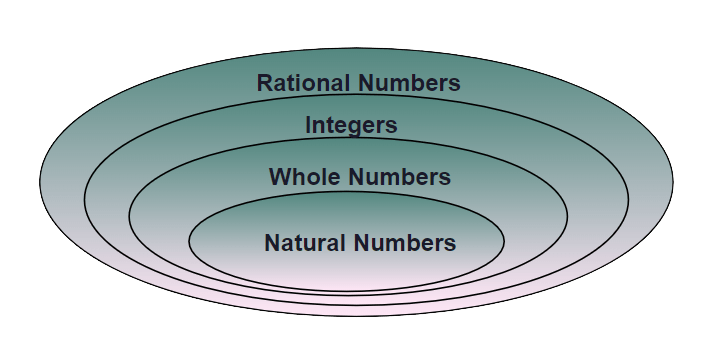### Rational Number List

The list of all rational numbers has been listed below:

• All the Natural Numbers are Rational Numbers
• All the Whole Numbers are Rational Numbers
• All the integers are Rational Numbers
• All the Fractions are Rational Numbers
• All the Terminating Decimals are Rational Numbers
• All the Non-Terminating and Repeating Decimals are Rational Numbers.
• The square roots of all the perfect squares are Rational Numbers
• The Cube Roots of all the perfect cubes are Rational Numbers.

## Decimal Expansion of Rational Number

There are two decimal forms of Rational Numbers, terminating and recurring.

### Terminating Decimal

A rational number whose decimal expansion has finite terms after decimal is called a terminating decimal. For Example, 1/4 = 0.25, 8/5 = 1.6 etc. A rational number has terminating decimal expansion if the denominator is in the form of 2m ⨯ 5n where m and n can be zero. Terminating Decimal Examples include 1/4 = 0.25, 25/4 = 6.25, 1/2 = 0.5 etc.

### Non-Terminating and Repeating(Recurring) Decimal

A rational number whose decimal expansion is non-terminating decimals i.e. which does not have finite terms after decimals and the repeating of digits is periodic i.e. repeat after the fixed interval is called Non-Terminating and Repeating Decimals. They are also called Recurring Decimals. A rational number which is not in the form of 2m ⨯ 5n where m and n can be zero i.e. it may be some other prime factors or maybe some extra in addition to 2 and 5 then the expansion is non-terminating and repeating. Examples of Recurring Decimals are 1/3 = 0.333…, 1.232323…

## Difference Between Fractions and Rational Numbers

Fractions are the real numbers represented in the form of a/b where both a and b are whole numbers whereas rational numbers are the real numbers represented in the form of a/b where both a and b are integers. However, in both cases, the denominator should not be equal to 0. Thus, we can say that all fractions are rational numbers but rational numbers are not fractions.

## Operations on Rational Numbers

There are four most common operations for Rational Numbers, which are as follows:

• Subtraction
• Multiplication
• Division

The addition of two rational numbers can be done using the following step-by-step method where the addition of 3/4 and 1/6 is explained as an example.

Step 1: Find the common denominator (LCD) for both the rational number. i.e.,

The common denominator for 4 and 6 is 12.

Step 2: Convert both the rational number to equivalent fractions using the common denominator. i.e.,

3/4 = (3 x 3)/(4 x 3) = 9/12

and 1/6 = (1 x 2)/(6 x 2) = 2/12

Step 3: Add the numerators of the equivalent fractions obtained in step 2. i.e.,

9/12 + 2/12 = (9 + 2)/12 = 11/12

Step 4: Simplify the resulting fraction if possible. i.e.,

11/12 is already in its simplest form.

Thus, Addition of 3/4 and 1/6 is 11/12 .

### Subtraction of Rational Numbers

Subtraction of two Rational Numbers can be done using the following step-by-step method where subtraction of 1/3 and 2/5 is explained.

Step 1: Find the common denominator (LCD) for both the rational number. i.e.,

The common denominator for 3 and 5 is 15.

Step 2: Convert both the rational numbers to equivalent fractions with the common denominator. i.e.,

1/3 = (1 x 5)/(3 x 5) = 5/15

and 2/5 = (2 x 3)/(5 x 3) = 6/15

Step 3: Subtract the numerators of the equivalent fractions obtained in step 2. i.e.,

5/15 – 6/15 = (5 – 6)/15 = -1/15

Step 4: Simplify the resulting fraction if possible. i.e.,

-1/15 is already in its simplest form.

Therefore, 1/3 – 2/5 = -1/15.

### Multiplication of Rational Numbers

Multiplication of two rational numbers can be achieved by simply multiplying the numerator and denominator of the given Rational Numbers. Step by step method with an example of multiplication of -11/3 and 4/5 is as follows:

Step 1: Write both rational number in with multiplication sign(×) in between. i.e.,

-11/3 × 4/5

Step 2: Multiply the numerator and denominator individually. i.e.,

(-11 × 4)/(3 × 5)

Step 3: We get the result of the multiplication. i.e.,

-44/15

### Division of Rational Numbers

Division of two Rational numbers can be achieved in the following steps(where the division of 3/5 and 4/7 is explained):

Step 1:  Write both rational number in with division sign in between. i.e.,

3/5 ÷ 4/7

Step 2: Change “÷” with  “×” and take raciprocal of the second rational number. i.e.,

3/5 × 7/4

Step 3: Multiply the numerator and denominator of the resulting fractions. i.e.,

(3 × 7)/(5 × 4)

Step 4: We get the result of the division. i.e.,

21/20

The image added below shows all the properties of the rational numbers.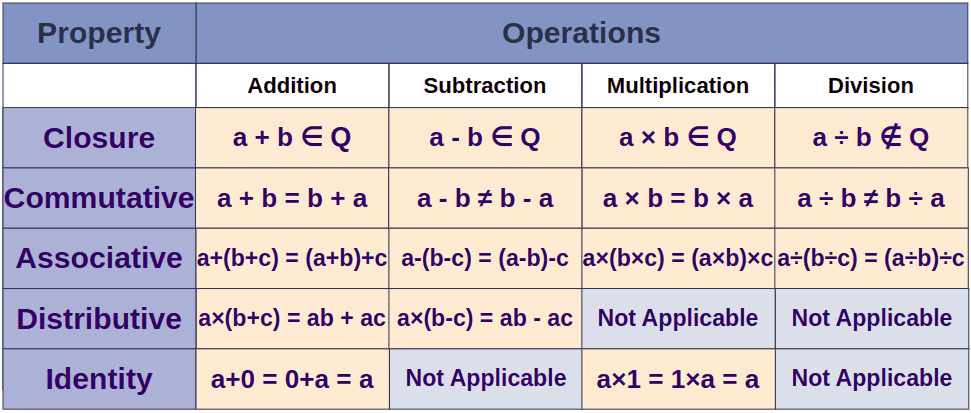## Rational Numbers Properties

Rational Numbers show several properties under the different operations (two of such common operations are addition and multiplication), which are as follows:

• Closure Property
• Commutative Property
• Associative Property
• Identity Property
• Inverse Property
• Distributive Property

### Closure Property

• Closure Property for Addition: Rational numbers are closed under addition, i.e., for any two rational numbers a and b, the sum a + b is also a rational number.
• Closure Property for Multiplication: Rational numbers are closed under multiplication, i.e., for any two rational numbers a and b, their product ab is also a rational number

Example:

For a = 3 / 4 and b = (-1) / 2

Now,  a + b = 3 / 4 + (-1) / 2

⇒ a + b = (3- 2)/ 4

⇒ a + b = 1/4, is Rational Number.

Also, a × b = 3/4 × (-1)/2 = -3/8,  which is also Rational number.

### Commutative Property

• Commutative Property for Addition: Rational numbers hold commutative property under addition operation, i.e., for any two rational numbers a and b, a + b = b + a.
• Commutative Property for Multiplication:  Rational numbers hold commutative property under multiplication operation as well, i.e., for any two rational numbers a and b, ab = ba.

Example: For a = (-7) / 8 and b = 3 / 5, verify commutative property.

Now, a + b = -7/8 + 3/5

⇒ a + b = (-7 x 5 + 3 x 8)/40 = (-35 + 24) / 40

⇒ a + b = (-11) / 40

And, b + a = 3/5 + (-7)/8

⇒ b + a = (3 x 8 + (-7) x 5)/ 40  = (24 – 35)/40

⇒ b + a =  -11/40 = a + b

Now, ab = (-7)/8 x 3/5 = (-7 x 3)/(8 x 5)

⇒ ab = -21/40

And, ba = 3/5 x (-7)/8 = (3 x 7 )/(5 x 8)

⇒ ba =(-21)/40 = ab

### Associative Property

• Associative Property for Addition: Rational Numbers are associative under addition operation, i.e., for any three Rational Numbers a, b, and c, a + (b + c) = (a + b) + c
• Associative Property for Multiplication: Rational Numbers are associative under multiplication operation as well, i.e., for any three Rational numbers a, b, and c, a(bc) = (ab)c

Example: For three Rational numbers a,b,c where a = -1/2, b = 3/5, c = -7/10, verify associative property.

Now, a + b = -1/2 + 3/5 = (-5 + 6)/10 = 1/10

and (a + b) + c = 1/10 + (-7)/10

⇒ (a + b) + c = (1 – 7)/10 = -6/10 = -3/5

Also, b + c = 3/5 + (-7)/10

⇒ b + c = (6 – 7)/10 = -1/10

and, a + (b + c) = -1/2 + (-1)/10

⇒ a + (b + c) = (-5 – 1)/10= -6/10 = -3/5

Thus, (a + b) + c = a + (b + c) is true for Rational Numbers.

Similarly, for multiplication

a × b = -1/2 × 3/5 = -3/10

and, (a × b)× c = -3/10 × -7/10= -3× (-7)/100

⇒ (a × b)× c  = 21/100

Also, b× c = 3/5 × (-7)/10 = -21/50

and, a × ( b × c ) = -1/2 × (-21)/50

⇒ a × ( b × c ) = 21/100

Thus, (a× b)× c = a × ( b × c ) is true for Rational Numbers.

### Identity Property

• Identity Property for Addition: For any rational number a, there exists a unique rational number 0 such that 0 + a = a = a + 0, where 0 is called the identity of the rational number under the addition operation.
• Identity Property for Multiplication: For any rational number a, there exists a unique rational number 1 such that a × 1 = a = a × 1, where 1 is called the identity of the rational number under the multiplication operation.

### Inverse Property

• Additive Inverse property: For any rational number a, there exists a unique rational number -a such that a + (-a) = (-a) +  a = 0, and -a is called the inverse of element a under the operation of addition. Also, 0 is the additive identity.
• Multiplicative Inverse property: For any rational number b, there exists a unique rational number 1/b such that b × 1 / b = 1 / b × b = 1, and 1/b is called the inverse of the element b under the multiplication operation. Here, 1 is the multiplicative identity.

Example:

For a = -11/23
a + (-a) = -11/23 – (-11)/23
a + (-a) = -11/23 + 11/23 = (-11 + 11)/23 = 0

Similarly, (-a) + a = 0

Thus, 11/23 is the additive inverse of -11/23.

Now, for b = -17/29
b × 1/b = -17/29 × -29/17 = 1

Similarly, 1 / b × b = 29/17 × -17/29 = 1

Thus, -29 / 17 is the multiplicative inverse of -17/23.

### Distributive Property

Distributive property for any two operations holds if one distributes over the other. For example, multiplicative is distributive over addition for the collection of rational numbers, for any three rational numbers a, b, and c the distributive law of multiplication of addition is

a × (b +c) = (a× b) + (a × c), and it is true for all the rational numbers.

Example: For rational number a, b, c i.e., a = -7 / 9, b = 11 / 18 and c = -14 / 27, verify distributive.

Now, b + c = 11/18 + (-14)/27
⇒ b + c = 33/54 + (-28)/54 = (33 – 28)/54 = 5/54

and, a × ( b + c ) = -7/9 × 5/54
⇒ a × ( b + c ) = (-7 × 5)/(9 × 54) = -35/486 . . .(1)

Also, a × b = -7/9 × 11/18
⇒ a × b = (-7 × 11)/9 × 18 = -77/9 × 9 × 2

and a × c = (-7)/ 9 ×(-14)/27
⇒ a × c = (7 × 14)/9 × 9 × 3 = 98/9 × 9 × 3

Now, (a × b) + (a × c) = (-77/9 × 9 × 2 ) + ( 98/9 × 9 × 3)
⇒ (a × b) + (a × c) = (-77 × 3 + 98 × 2)/9 × 9 × 2 × 3
⇒ (a × b) + (a × c) = (-231 + 196)/486 = -35/486 . . .(2)

(1) and(2) shows that  a × ( b + c ) = ( a × b ) + ( a × c ).

Hence, multiplication is distributive over addition for the collection Q of rational numbers.

## Rational Number between two given Rational Numbers

Between two rational numbers there exists infinite rational numbers. However, we can find a rational number between two rational numbers using the formula 1/2(a + b) where a and b are rational numbers. Let’s say we have to find rational numbers between 2 and 3 then a rational number between 2 and 3 is given as 1/2(2 + 3) = 5⨯1/2 = 5/2

However, other methods also exist to find rational numbers between two rational numbers.

### Method 1: To find rational numbers between two rational numbers with like denominators.

In this, we need to multiply the numerator and denominator of rational numbers with a larger number to create a gap between numerators.

Once the gap is created write the in-between rational numbers just increasing the numerator by 1 and keeping the denominators same.

Example: Find 10 rational numbers between 4/5 and 6/5.

Solution:

In this case, we see that we can only find only one rational number between 4/5 and 6/5 which is 5/5. But here we need to find 10 rational numbers.

Hence, we would multiply the numerator and denominator in both the rationals by 10. Hence we have to find 10 rational numbers between 4⨯10/5⨯10 and 6⨯10/5⨯10 i.e. 40/50 and 60/50.

Hence, ten rational numbers between 40/60 and 50/60 are 41/50, 42/50, 43/50, 44/50, 45/50, 46/50, 47/50, 48/50, 49/50, 50/50.

If we need more we would multiply by a larger number. For simplicity, you can multiply by 10, 100, etc.

### Method 2: To find a rational number between two rational numbers with unlike denominators

In this, we first convert the unlike denominators to like decimals then follow the same method as followed in the case of like denominators

Example: Find 5 rational numbers between 4/3 and 6/5

Here we will first make the denominators like, by taking the LCM of denominators 3 and 5. Hence, the LCM of 3 and 5 is 15. Therefore our new equivalent rational numbers will be 4⨯5/3⨯5 and 6⨯3/5⨯3 i.e. 20/15 and 18/15.

Still, we see that gap is of two only between 18 and 20. Hence, we will multiply with a larger number say 5.

Hence, we have to find a rational number between 20⨯5/15/⨯5 and 18⨯5/15⨯5 i.e. 100/75 and 90/75. Hence, 5 rational numbers between 90/75 and 100/75 are 91/75, 92/75, 93/75, 94/75 and 95/75.

### Method 3: To find ‘n’ rational numbers between two rational numbers x and y with unlike denominators such that x < y

In this case, first calculate, d = (y – x)/(n + 1) then find the rational numbers between two rational numbers as (x + d), (x + 2d), (x + 3d),…..,(x + nd)

Example: Find five rational numbers between 1/3 and 2/5.

x = 1/3, y = 2/5, n = 5

d = (y – x)/(n + 1) = (2/5 – 1/3)/(5 + 1) = 1/15/6 = 1/90

Five rational numbers between 1/3 and 2/5 are given as

(x + d), (x + 2d), (x + 3d), (x + 4d) and (x + 5d)

(1/3 + 1/90), (1/3 + 2/90), (1/3 + 3/90), (1/3 + 4/90) and (1/3 + 5/90)

(31/90), (32/90), (33/90), (34/90) and (35/90)

## Representing Rational Number on Real Line

Rational Numbers are Real Numbers. Hence they can be represented on real line. There are following steps that need to be followed to represent rational numbers on real line.

Step 1: First find if the number is positive or negative if positive then it will be plotted on the RHS of zero and if positive it will be on the LHS of zero.

Step 2: Identify if the given rational number is proper or improper. If proper then it will lie between 0 and 1 in case of positive and 0 and -1 in case of negative rational number.

Step 3: If improper then convert it into a mixed fraction. In this case, the rational number will lie just beyond the whole number part.

Step 4: Now after steps 1, 2, and 3 we have to plot only the proper fraction part. To plot this cut the area between the two successive desired numbers by drawing lines n-1 times where n is the denominator of the proper fraction part.

Step 5: Now count the lines equal to the value of the numerator. This will represent the desired rational number on a real line.

Let’s see some examples:

Example 1: Represent 2/5 on Real Line

Solution: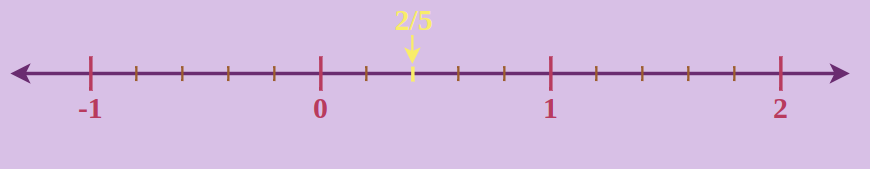Example 2: Represent -2/5 on Real Line

Solution: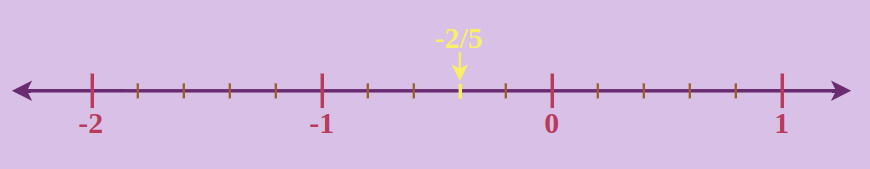Example 3: Represent 4/3 on Real Line

Solution:

4/3 = 1(1/3)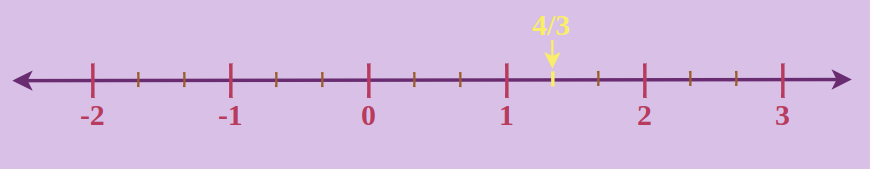Example 4: Represent -4/3 on Real Line

Solution:

-4/3 = -{1(1/3)}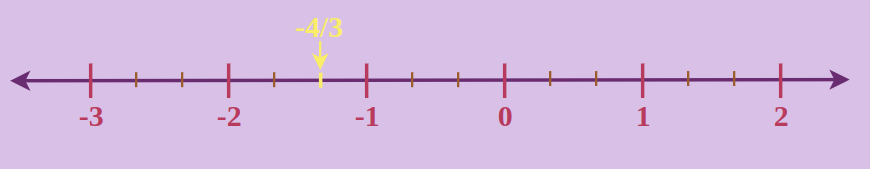## Rational and Irrational Numbers

Irrational Numbers are those which can’t be represented in the form of p/q where q ≠ 0. The decimal expansion of irrational numbers is non-terminating and non-repeating. Let’s learn a comparison between the rational and irrational numbers in the table given below:

Rational Numbers

Irrational Numbers

It can be represented in the form of p/q where q ≠ 0

It can’t be represented in the form of p/q where q ≠ 0

Its Decimal Expansion is either terminating or non-terminating and repeating

Its Decimal Expansion is non-terminating and non-repeating

A set of rational numbers contains all types of numbers such as natural numbers, whole numbers, and integers.

Irrational Numbers doesn’t contain all types of numbers in itself

Examples include 2/3, -5/6, 0.25, 0.333, 22/7, etc.

Examples include √2,√3, 1.010010001, π, etc.

## Solved Examples on Rational Numbers

Example 1: Check which of the following is irrational or rational: 1/2, 13, -4, √3, and π.

Solution:

Rational numbers are numbers that can be expressed in the form of p/q, where q is not equal to 0.

1/2, 13, and -4 are rational numbers as they can be expressed as p/q.

√3, and π are irrational numbers as they can not be expressed as p/q.

Example 2: Check if a mixed fraction, 3(5/6) is a rational number or an irrational number.

Solution:

The simplest form of 3(5/6) is 23/6

Numerator = 23, which is an integer

Denominator = 6, is an integer and not equal to zero.

So, 23/6 is a rational number.

Example 3: Determine whether the given numbers are rational or irrational.

(a) 1.33  (b) 0.1  (c) 0  (d) √5

Solution:

a) 1.33 is a rational number as it can be represented as 133/100.

b) 0.1 is a rational number as it can be represented as 1/10.

c) 0 is a rational number as it can be represented as 0/1.

d) √5 is an irrational number as it can not be represented as p/q.

Example 4: Simplify (2/3) × (6/8) ÷ (5/3).

Solution:

(2/3) × (6/8) ÷ (5/3) = (2/3) x (6/8) × (3/5)

= (2 × 6 × 3)/(3 × 8 × 5)

= 36/120 = 3/10

Example 5: Arrange the following rational numbers in ascending order:

1/3, -1/2, 2/5, and -3/4.

Solution:

The common denominator for 3, 2, 5, and 4 is 60. Thus

1/3 = 20/60,
-1/2 = -30/60,
2/5 = 24/60,
-3/4 = -45/60

With common denominator, rational number with greatest numerator is greatest.

⇒ -30/60 < -45/60 < 20/60 < 24/60
Thus, ascending order of given rational numbers is: -1/2 < -3/4 < 1/3 < 2/5

## FAQs on Rational Numbers

### 1. What are Rational Numbers?

A rational number is a number that is in the form of p/q, where p and q are integers, and q is not equal to 0. Some examples of rational numbers include 1/3, 2/4, 1/5, 9/3, and so on.

### 2. What is the Difference between Rational and Irrational Numbers?

A rational number is a number that is expressed as the ratio of two integers, where the denominator should not be equal to zero, whereas an irrational number cannot be expressed in the form of fractions. Rational numbers are terminating decimals but irrational numbers are non-terminating and non-recurring. An example of a rational number is 10/2, and an irrational number is a famous mathematical value Pi(π) which is equal to 3.141592653589…….

### 3. Is 0 a Rational Number?

Yes, 0 is a rational number because it is an integer that can be written in any form such as 0/1, 0/2, where b is a non-zero integer. It can be written in the form: p/q = 0/1. Hence, we conclude that 0 is a rational number.

### 4. Is Pi(π) a Rational Number?

No, Pi (π) is not a rational number. It is an irrational number and its value equals 3.142857…

### 5. Are Fractions Rational Numbers?

Fractions are numbers that are represented in the form of (numerator/denominator) which is equivalent to p/q form so fractions are considered rational numbers. Example 3/4 is a fraction but is also a rational number.

### 6. Are all Rational Numbers Integers?

No, all rational numbers are not integers but the opposite is true. i.e. “all integers are rational numbers.” For example, 1/2 is a rational number but not an integer whereas -7 is an integer and is also a rational number.

### 7. Can Rational Numbers be Negative?

Yes, a rational number can be negative i.e. all negative number comes under rational numbers. Example -1.25 is a rational number.

### 8. Are all Whole Numbers Rational Numbers?

Yes, all whole number are considered as rational numbers. For example 1 is a whole number and is also a rational number.

### 9. How many Rational Numbers are between 1/2 and 1/3?

There are infinite rational numbers between any two rational number, thus there are infinite rational numbers between 1/2 and 1/3, some of those numbers are 11/24, 7/24, 19/48, 13/72, 3/8 etc.

### 10. By which Symbol Rational Number denoted?

Rational Numbers are denoted by “Q” in the mathematics.

### 11. How many Rational Numbers are there?

There are infinitly many rational numbers.

Whether you're preparing for your first job interview or aiming to upskill in this ever-evolving tech landscape, GeeksforGeeks Courses are your key to success. We provide top-quality content at affordable prices, all geared towards accelerating your growth in a time-bound manner. Join the millions we've already empowered, and we're here to do the same for you. Don't miss out - check it out now!

Previous
Next